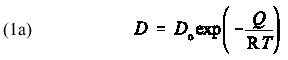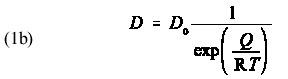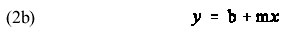Graphical Representation

One technique for helping students understand the role of variables and parameters in an equation is to explore graphical representations. Several computer programs are commercially available for this purpose including Matlab, Mathmatica, Genplot, or Excel . (Tutorials are available for some of these software products.) We illustrate this point with two examples. (PDF version (Acrobat (PDF) 886kB Jul17 04) of this discussion.)

Example 1:

The rate of diffusion of atoms or ions through a rock is described by a diffusion coefficient, D, a parameter that increases exponentially with increasing temperature, T. The diffusion coefficient is expressed as an Arrhenius equation of the formwhere the pre-exponential factor, D is material-dependent parameter, Q is the activation energy, and R is the gas constant. It may not be obvious from this equation that D increases with increasing temperature. Therefore, I ask students to use their calculators to help me make a plot of D versus 1/T on the blackboard using Q = 150 kJ/mol and D = 1 m /s with R = 28.314 kJ/mol/K. After plotting D versus T on the blackboard, I hand out a hard copy of Figure 1. From this graph, it is clear that D indeed increases with increasing T. In addition, I find it valuable to discuss the behavior of D in the limits that T goes to zero and T goes to infinity. Figure 1 illustrates that D goes to zero as T goes to 0 and that D goes to infinity at T goes to infinity. [Aside: It may be helpful to ask students to compare values of D/D for the case in which Q/RT = 1 with the case in which Q/RT = 2, noting that the first case corresponds to a lower temperature than the second case. With this approach, I find it useful to rewrite Equation (1a) asso that the students are comparing 1/e (low temperature) with 1/e2 (high temperature).]

Because the dependence of D on T is exponential in form, it is instructive for the students if you rewrite Eq. (1a) aswhich is a linear equation of the usual formI again ask the students to use their calculators to help me plot the diffusion data on a semi-log graph to emphasize the linear relationship between ln(D) and 1/T, as illustrated in Figure 2. To complete this part of the exercise, I point out that if they were given a set of diffusion data such as shown in Figure 3, they can determine the activation energy from the slope, since the slope m = -Q/R such that Q = -m/R. Likewise, they can determine D0 from the intercept, since b = ln(D0) such that D0 = exp(b).